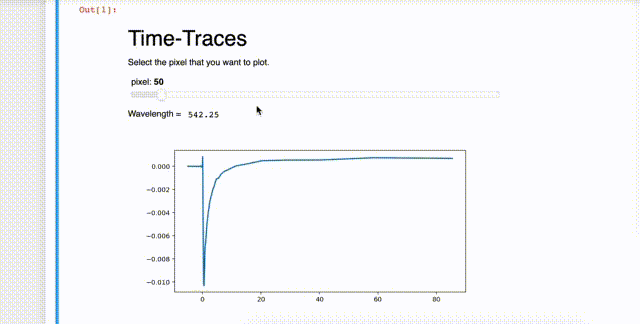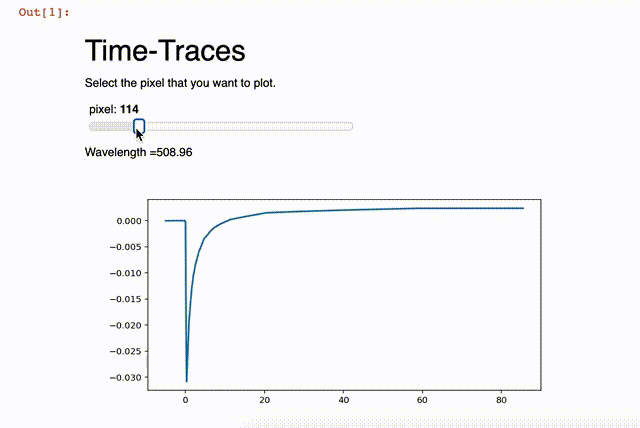# Slider for a figure can be used to update an integer value on the dashboard?

Hello everyone,

First of all, thanks for this great app. I started building my own data analysis tool with Panel. My question is as in the title. I want to do something very simple. I have a slider for a figure. It is an input parameter, `pixel`, just slicing (or indexing) my 2-dimensional array for single plots. However, every pixel value (arbitrary) corresponds to a wavelength value which lies in the first row of my data. I want that slider “pixel” also represent and update that wavelength value on dashboard next to the figure. I tried using `i.value` to get the value of the pixel slider, and calculate `wavelength` but function is not catching the value when I move the slider. How can I achieve this?Any idea or direction would be highly appreciated! Thanks!Here is the code that I am working on.

``````import pandas as pd
import numpy as np
import panel as pn
import matplotlib.pyplot as plt
from matplotlib.figure import Figure
from matplotlib.backends.backend_agg import FigureCanvas

#Global Variables
fig_width = 8
fig_height = 4

%matplotlib inline
%matplotlib notebook

d = np.genfromtxt('visdata.dat', delimiter='\t')

def plot_timtrace(pixel=50):
fig = Figure(figsize=(fig_width, fig_height))
ax = fig.subplots()
x1=(d[7:50,0]) #slicing first column of data as x-axis (which is time)
y1=(d[7:50,pixel]) #slicing second column of data as y-axis (which is 1st pixel..)
ax.plot(x1, y1, linewidth=2.0)
return fig

import panel as pn
pn.extension()

pn.interact(plot_timtrace)

kw = dict(pixel=(1, (d.shape)-1))
i = pn.interact(plot_timtrace, **kw)

text = "<br>\n#Time-Traces\nSelect the pixel that you want to plot."

def find_wl():
wl = d[0,i.value]
return(wl)

wavelen_text = pn.interact(find_wl)

p = pn.Column(pn.Row(text) ,i, pn.Row("Wavelength =", wavelen_text), i)
p``````

Hi,

A quick way to do this would be:

``````import pandas as pd
import numpy as np
import panel as pn
import matplotlib.pyplot as plt
from matplotlib.figure import Figure
from matplotlib.backends.backend_agg import FigureCanvas

pn.extension()

#Global Variables
fig_width = 8
fig_height = 4

# %matplotlib inline
# %matplotlib notebook

d = np.genfromtxt('visdata.dat', delimiter='\t')

#d =np.random.rand(50,600)  # don't have your data - I'm guessing the data looks something like this?
#d[7:50,0] = 2*np.arange(43) # index not random

def plot_timtrace(pixel=50):
fig = Figure(figsize=(fig_width, fig_height))
ax = fig.subplots()
x1=(d[7:50,0]) #slicing first column of data as x-axis (which is time)
y1=(d[7:50,pixel]) #slicing second column of data as y-axis (which is 1st pixel..)
ax.plot(x1, y1, linewidth=2.0)
return pn.Column(f'Wavelength ={d[0,pixel]}',fig)

wavelen_text = pn.interact(plot_timtrace,
pixel=range(1,d.shape))

pn.Column("<br>\n#Time-Traces\nSelect the pixel that you want to plot.",wavelen_text)
``````
1 Like

Hi Mats,

Thanks a lot! I tested it quickly and it works!I had actually tried something similar to your solution but did not get it to work. Because i was returning the function as below;

``````return (wavelength, fig)
``````

Which was not plotting the figure but only returning the wavelength variable. Why do I need to capture them in `pn.Column` ?

In order to use `pn.interact` you need to return an object Panel can display like a `pn.Column` or some plot object or even text. Returning a tuple doesn’t work.

1 Like